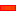Dyads [also known as Assur Groups] always have:

 • Two Parts
 • Three Joints

There is no limit to the number of Dyads in a single kinematic-chain.

The joints specify the relative motion of the Parts in the Dyad.

A Dyad, or Assur group, is a kinematic chain with zero degree of mobility, which when added to or subtracted from a kinematic-chain does not alter its original degrees-of-freedom.

They were first described by the Russian engineer Leonid Assur [es] (1878-1920) in 1914.

####Dyads: Degrees-of-Freedom

 The Gruebler Equation shows that a Dyad has Zero Degrees-of-Mobility. Gruebler Equation: F = 3*(N-1) – 2*J1 – J2 F = 3 * (3-1) – 2*3 –0 F = 6 – 6 F= 0 N = 3 There are two Parts in a Dyad. You must join these two other Parts. However, when they are already kinematically-defined, you, and the Gruebler equation, take them to be one Part. Hence N= 2+1 =3 J1 = 3 There are three joints J2 = 0 There are not any higher pairs [e.g' Cams and Follower Joint which has two degrees of freedom] Therefore, a Dyad does not change the degrees-of-freedom of a kinematic-chain. It also means you can add as many Dyads as you want to a kinematic-chain. The number of Degrees-of-Freedom does not change.

####Dyads: The Basic Construction

We do not identify a Dyad by the number of its Parts. This is because ALL dyads have two Parts.

We identify a Dyad with three letters, because ALL dyads have three Joints.

 • Each letter represent a Joint in the Dyad.
 • The letters are R, P and S.
 • Why R, P and S?
 o R is for Revolute Joint. This is the same as a Pin-Joint.
 o P is for Prismatic Joint. This is the same as a Slide-Joint.
 o S is for Spherical Joint. This is the same as a Ball-Joint

We can re-write the dyad with dashes[—] between each letter so that the dash[—] represents a Part.

Then, it is easier to see that the middle letter represents the joint with which the two Parts are joined together.

The other two letters represent the joints with which the two Parts are joined to two other Parts in the kinematic-chain.

If the kinematic-chain is a kinematically-defined chain BEFORE you add a new dyad, it will also be kinematically-defined chain AFTER you add the dyad.

KDP1 > {<JOINT1> PART1 <JOINT2> PART2 <JOINT3>} < KDP2

KDP1, KDP2 : Kinematically Defined Parts

PART1, PART2 : Parts in the Dyad

JOINT, JOINT, JOINT3 : Joints in the DyadPreparation: Two different Kinematically Defined Parts that already exist in the model

 • A Rocker(KDP1) joined to the Base-Part(KDP2).Part1and Part2Joint1, Joint2, Joint3Joint1& Joint3are the 'first and last' Joints.

These join the two Parts in the Dyad to the Kinematically Defined Parts (KDP1 & KDP2) that already exist in the model.Joint2is the 'middle' Joint.

It joins the two Parts in the Dyad together.

####Dyads: Suggested Steps to Add a new DyadSTEP 1: Start with a Kinematic-Chain with a minimum of two Kinematically Defined Parts: here a Rockerand Base-PartSTEP 2: Add a Two new Parts - Part1and Part2STEP 3: Add 'Outside' Joint1between Part1and Kinematically Defined Part1STEP 4: Add 'Outside' Joint3between Part2and Kinematically Defined Part2STEP 5: Add 'Inside' Joint2between Part1and Part2####Dyads: in the Kinematic Tree

A Dyad [Assur Group] is identified with a Three Letter Acronym. Each letter represents a Joint. The letters we use are R, P and S.

Why R, P and S?

 • The letter R is a Revolute Joint. It is exactly the same as a Pin-Joint.
 • The letter P is a Prismatic Joint. It is exactly the same as a Slide-Joint.
 • The letter S is a Spherical Joint. It is exactly the same as a Ball-Joint.

There are five possible Dyads in Planar Kinematics:

Kinematic Name

MechDesigner Name

R R RRevolute – Revolute – Revolute

Pin – Pin – Pin

R R PRevolute – Revolute – Prismatic

Pin – Pin – Slide

R P RRevolute – Prismatic – Revolute

Pin – Slide – Pin

P R PPrismatic – Revolute – Prismatic

Slide – Pin – Slide

R P PRevolute – Prismatic – Prismatic

Pin – Slide – Slide

We have two 'Ram' type Dyads - useful for Air Cylinders

Ram - RRevolute – Revolute – Revolute

Pin +Motion-Point - Pin - Pin

Ram - PRevolute – Revolute – Prismatic

Pin +Motion-Point - Pin - Slide

We have two Dyads for Spacial Kinematics. More are possible but not frequently found in Packaging Machines.

Kinematic Name

MechDesigner Name

S S RSpherical – Spherical – Revolute

Ball – Ball – Pin

S S PSpherical – Spherical – Prismatic

Ball – Ball – Pin

Tutorial and Reference Help Files for MechDesigner and MotionDesigner 13.2 + © Machine, Mechanism, Motion and Cam Design Software by PSMotion Ltd